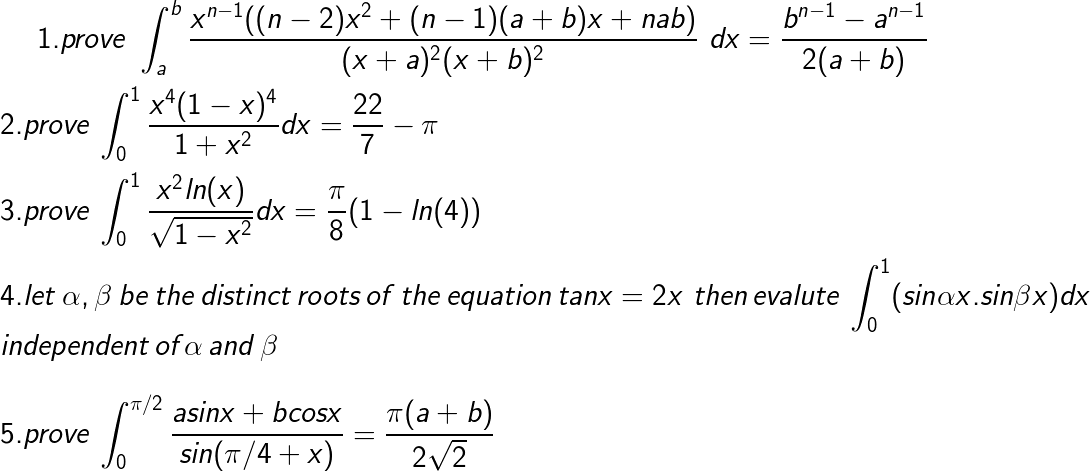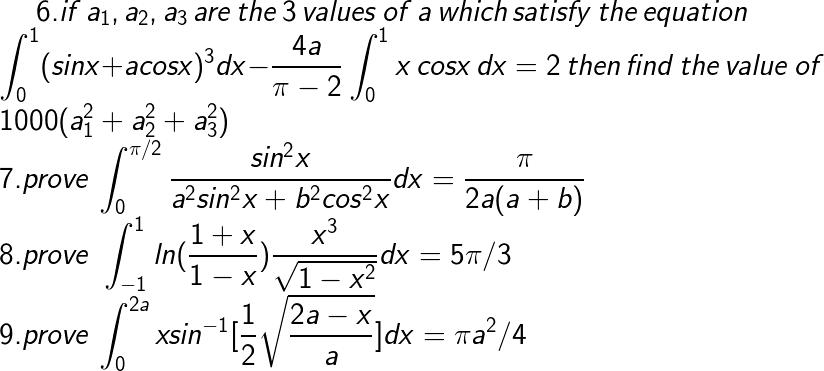# definite integral class sheet doubts•Amartya Kumar Mistry ·

for the fourth q one of the roots will be zero which makes the whole integral expression zero which is independent of Î± and Î²

Manish Shankar ·

First one is integral of differentiation of (xn(x+a)(x+b))

•Manish Shankar ·

second one is x8-4x7+6x6-4x5+x41+x2

divide it to simplify it

Manish Shankar ·

for question 6 change the intervals to (0->pi/2)

•Amartya Kumar Mistry ·

for q 5 sin[Ï€/2-(Ï€/4+x)]=sin(Ï€/4+x)
now apply the rule of definite integral âˆ«baf(x)=âˆ«abf(b+a-x) and also expand sin(x+Ï€/4) by trigonometry. then after simplifying a bit you will see that numer. and denom. is getting cancelled then putting the limits you will get the result.

•Amartya Kumar Mistry ·

for q 8 just apply the concept of integration by parts(LIATE rule) and there for the integration of x^3/âˆš(1-x^2) take x=sinÎ¸ as substitution

•